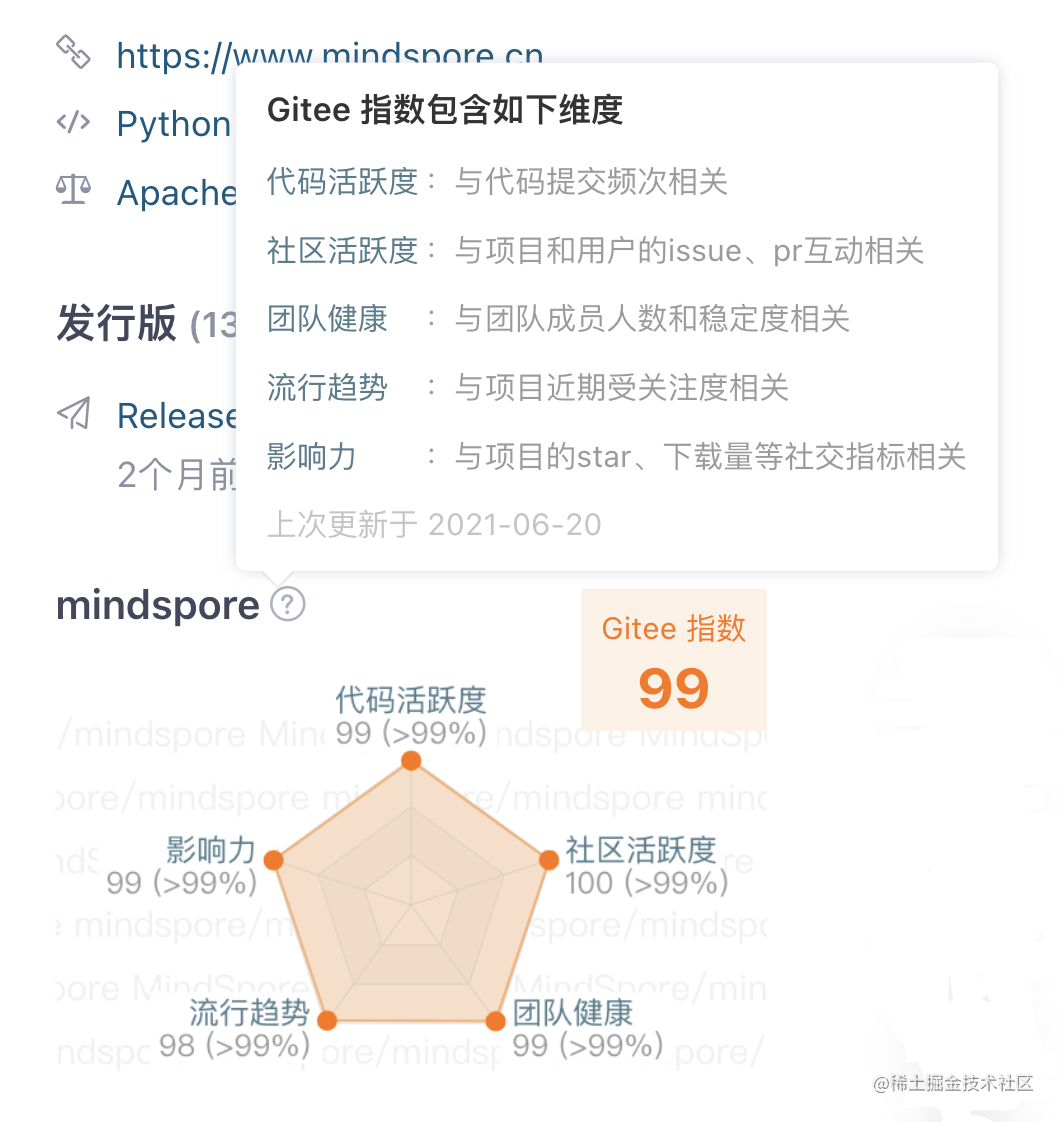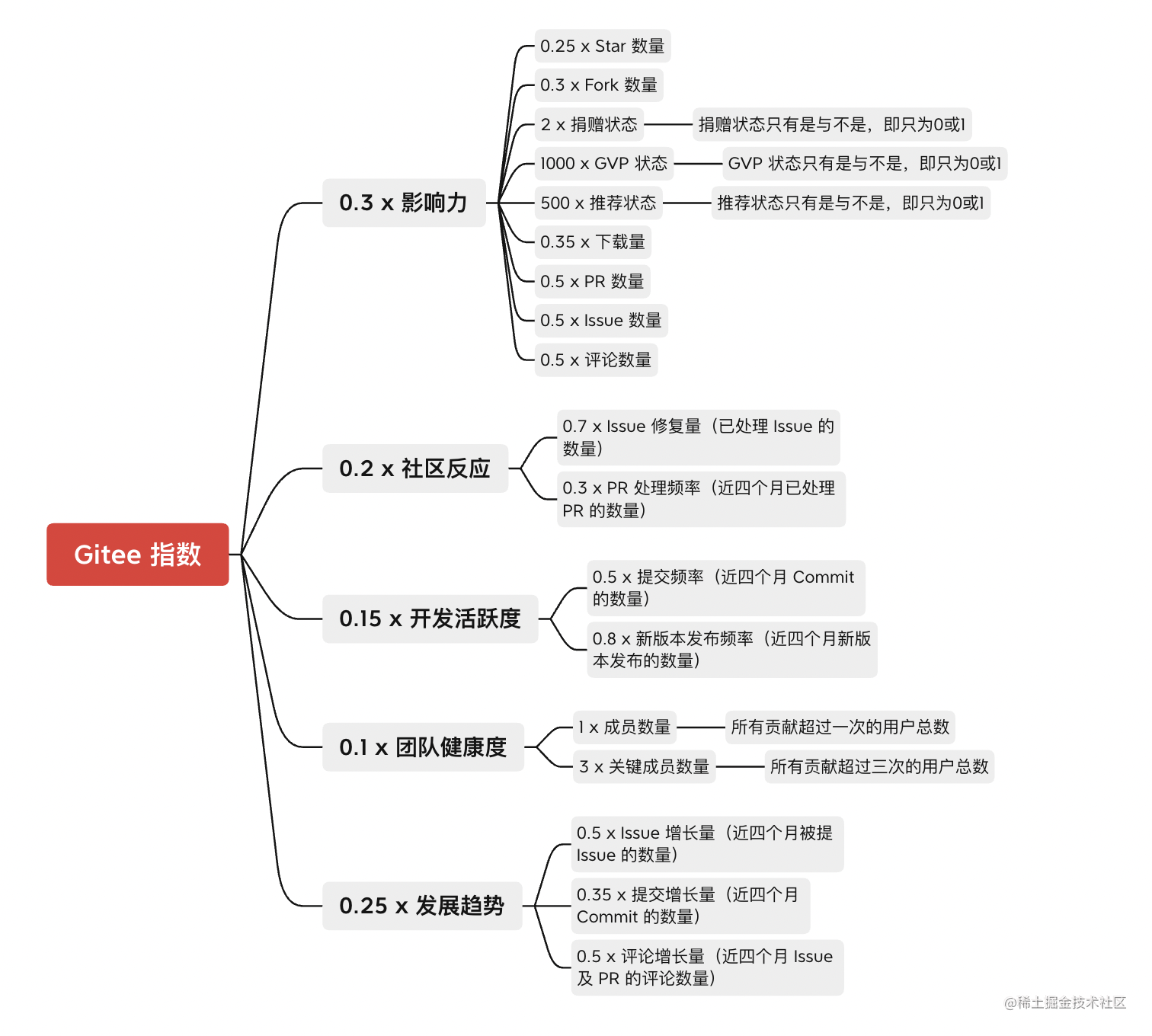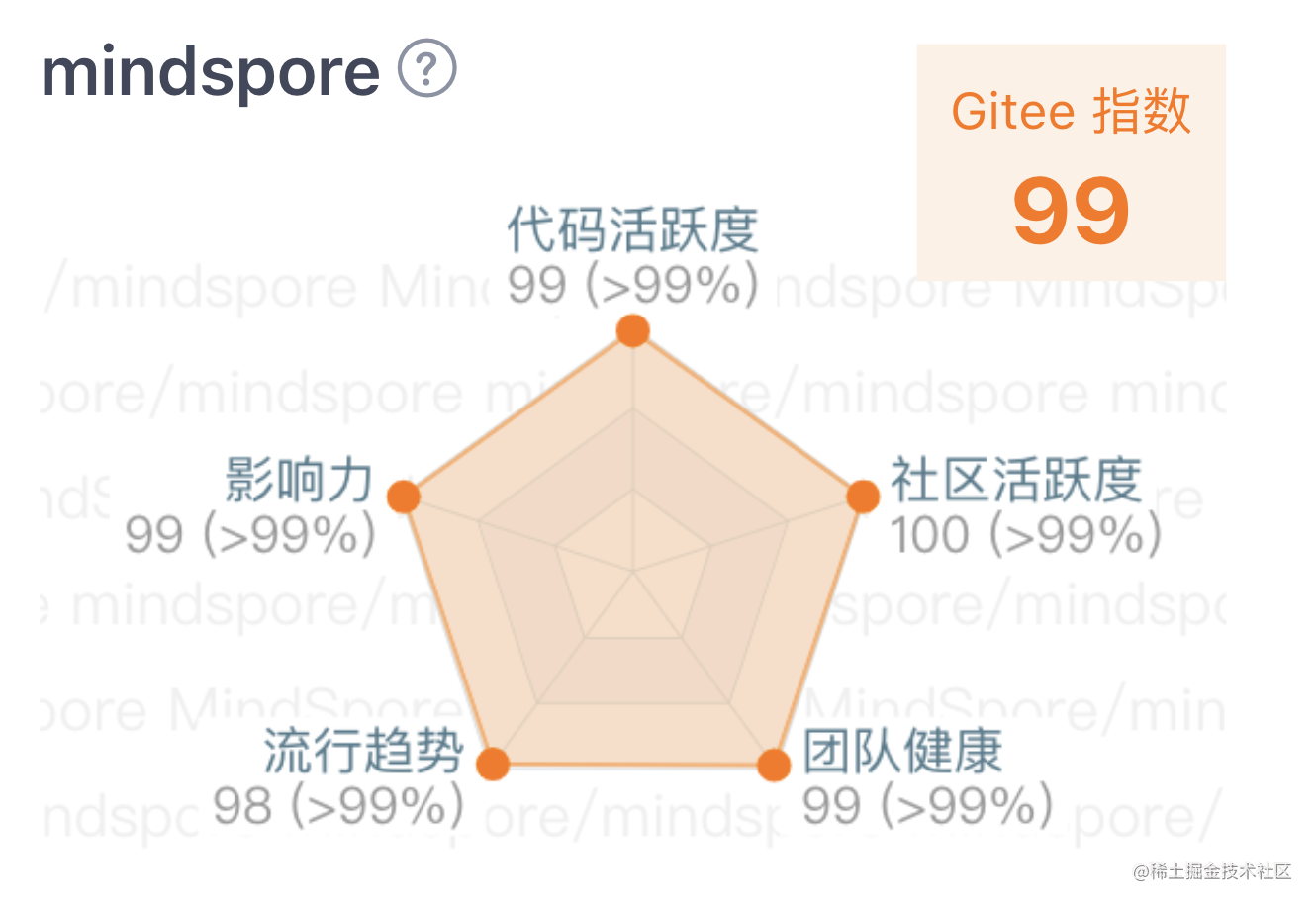# 还在看开源项目的Star？这个指标其实更值得关注！### Gitee指数评估维度

1.影响力

2.代码活跃度

3.社区活跃度

4.团队健康

5.流行趋势### 维度计算方法

$p=\sum_{i}^{} w_{i}f_{i}$

$p$为项目画像的维度， $fi$为维度下的各个分支特征，$wi$是基于业务场景中的经验权重。

$x^{*}=\frac{x-min(x_{i})}{max(x_{i})-min(x_{i})}$